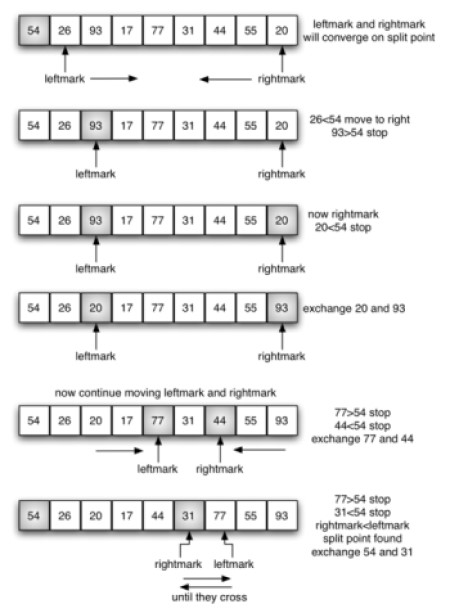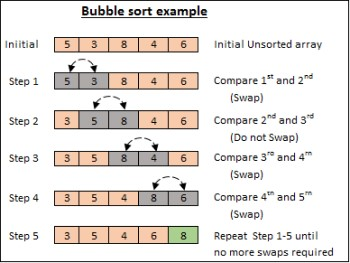# Difference between Quick Sort and Bubble Sort

##### Key Difference: Bubble sort is the simplest form of sorting algorithm technique that involves swapping of two adjacent elements in order to put them in right place, where as Quick sort works on split and win algorithm technique into which a pivotal element becomes the focal point of division around the given array.Quick Sort and Bubble Sort are two difference types of algorithms that are used for efficiently sorting data. Quicksort, also known as partition-exchange sort, is primarily used for placing the elements of an array in order. Whereas, bubble sort is a simple sorting algorithm that repeatedly steps through the list, compares adjacent pairs and swaps them if they are in the wrong order. It is also sometimes called a sinking sort.

While both sorting techniques are known to have a decent place in the computer science world, bubble sort is the simplest form of sorting algorithm technique that involves swapping of two adjacent elements in order to put them in right place, whereas Quick sort works on split and win algorithm technique into which a pivotal element becomes the focal point of division around the given array.

To understand these two concepts a little deeper, let’s break the differences into precise segmentation to make it clearer.

1. Approach: To have a clear idea let’s first differentiate on the basis of their algorithmic approach.

Bubble Sort: Let’s suppose there are 5 elements 9,5,3,6,1, and we need to sort them in ascending order.

1. 9 5 3 6 1  // first element check the adjacent element and swaps if bigger (here, 9>5)
2. 5 9 3 6 1 // (9>3)
3. 5 3 9 6 1 // (9>6)
4. 5 3 6 9 1 // (9>1)
5. 5 3 6 1 9 // 9 reached the final destination

Now, the next iteration begins:

1. 5 3 6 1 9 // (5>3)
2. 3 5 6 1 9 // (5<6)- No swapping
3. 3 5 6 1 9 // (6>1)
4. 3 5 1 6 9 // (6<9)- No swapping
5. 3 5 1 6 9 // 6 reached its final destination

--- Some more iterations---

The final end result would be

1 3 5 6 9 // all elements are finally sorted

Quick Sort: Let’s suppose, we have a bigger array of 7 numbers

1 3 8 9 4 5 7

We determine the pivotal number as 7, the last digit of the array.

Now 7 would be checked each time

1 8 3 9 4 5 7 // No swapping since it’s the first value

1 8 3 9 4 5 7 // No swapping since 8>7

1 3 8 9 4 5 7 // Swapping between 3 and 8 since 3<7

1 3 8 9 4 5 7 // No Swapping since 9>7

1 3 4 9 8 5 7 // Swapping between 4 and 8 since 4<7

1 3 4 5 8 9 7 // Swapping between 5 and 9 since 5<7

1 3 4 5 7 9 8 // Swapping between 7 and 8 since 9>7

Now since 7 has come to appropriate value by partitioning, we can perform the next step

1, 3, 4, 5, 7, 9, 8 //Since Quick is recursive we can call out for another partition of 1,3,4,5 and 9, 8.

1, 3, 4, 5 // 5 becomes is Pivot point, and checks every element

9, 8 // 8 becomes the pivotal point and checks the remaining elements

8, 9 // Swapping between 8 and 9 since 8<9.

Combining both we get our end-result

1, 3, 4, 5, 7, 8, 9

2. Time Complexity: Any algorithmic time complexity is generally denoted by the ‘big O notation’. The term ‘n’ is generally denoted the size of the array. The more the iterations are, the more time consumed. Thus, time complexity is determined by the total number of steps it takes to bring up the final result.

Bubble Sort has a time complexity of O(n^2), which means that the loop is exponentially increasing with increase in the value of n. If the value of n is 2, ideally the loop is going to run 4 times, and if it is 4, it would run 16 times; and so on… Thus, it would generate huge time issues when the value of n is large.

Quick Sort has a time complexity if O(n log n), which can possibly be less efficient  than normal techniques, still it yields much faster results.3. Coding: Undoubtedly, the creation of Bubble sort is one of the easiest sorting algorithm approach, as per any coder perspective. In fact, bubble sort is one of the first sorting techniques that coders are taught in order to introduce them to the sorting world.

Quick Sort, on the other hand, has a complex creation background. With the involvement of pivotal points and sub-algorithm that sorts the sub arrays, it becomes a little complex again.

4. Usefulness: During large arrays sorting, Bubble Sort performs poorly due to abundance of time consumption, thus it is mostly used for educational purpose, in order to make concepts of sorting easier to grasp for beginners. Still, it has a respectable place in sorting arrays for a lesser number of elements. Quick Sort is considered to be more useful in sorting as per industrial and production value, since its has an quicker and recursive results, especially when compared to Bubble Sort.

Comparison between Quick Sort and Bubble Sort:

 Quick Sort Bubble Sort Type Sorting Algorithm Sorting Algorithm Method Split and win algorithm technique into which a pivotal element becomes the focal point of division around the given array. Swaps of two adjacent elements in order to put them in right place Time Complexity O(n log n) O(n^2) Coding Complex Simpler Performance Recursive, Faster Slower, Iterative Time Consumption Less Time Consumption to run an algorithm More Time Consumption to run an algorithm Usefulness Considered to be more useful Considered to be less useful
```References: Wikipedia (Quicksort, Bubble Sort), Geeks for Geeks (Quick Sort, Bubble Sort),